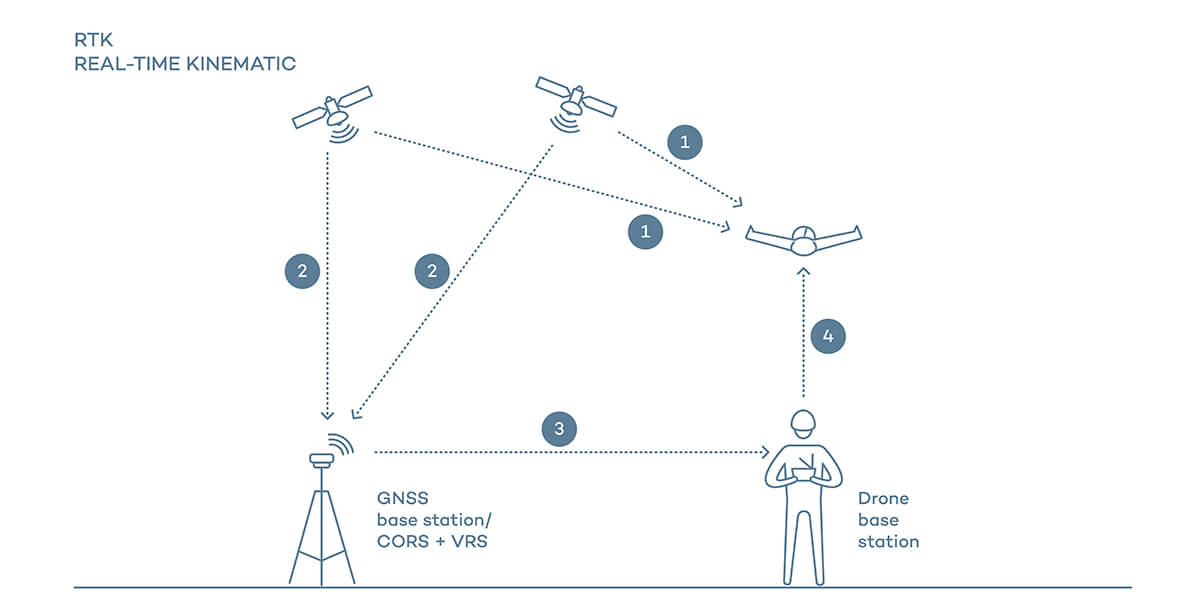# What factors will affect the precise navigation and positioning of drone?At present, most drones use a combination of GPS and inertial navigation for navigation. Inertial navigation usually uses two or more sensors (gyroscope, accelerometer, magnetic field meter and/or GPS) information for the aircraft relative to the earth. Heading vector and velocity vector. To understand what factors affect drone positioning, we first need to know how drone positioning works.

Accelerometers are used to measure acceleration. A three-axis accelerometer can measure the direction of motion of a stationary platform relative to the Earth's surface, but once the platform is in motion, the situation becomes much more complicated. If the platform is in free fall, the acceleration value measured by the accelerometer is zero. If the platform performs acceleration motion in a certain direction, each axial acceleration value will contain the acceleration value generated by gravity, making it impossible to obtain the real acceleration value. For example, a three-axis accelerometer mounted on an aircraft with a 60-degree roll angle would measure a vertical acceleration value of 2G, when in fact the aircraft was tilted 60 degrees relative to the surface of the area. Therefore, the use of the accelerometer alone cannot keep the aircraft in a fixed heading.

The gyroscope measures the angular rate of rotation of the body around a certain axis. When the gyroscope is used to measure the rotational angular rate of the axial direction of the aircraft body, if the aircraft is rotating, the measured value is non-zero, and when the aircraft is not rotating, the measured value is zero. Therefore, the roll angular rate value measured by the gyroscope on the aircraft with a roll angle of 60 degrees is zero, and the angular rate value is zero when the aircraft is flying horizontally and straightly. The current roll angle can be estimated by time integration of the angular rate values, provided there is no accumulation of errors. The value measured by the gyroscope will drift over time, and additional errors will accumulate over minutes or even seconds, which will eventually lead to a completely wrong perception of the current roll angle of the aircraft relative to the horizontal plane. Therefore, the gyroscope alone cannot maintain a specific heading of the aircraft.From this, it can be seen that the measurement value of the accelerometer in a longer period of time (determining the aircraft heading) is correct, but in a shorter period of time due to the existence of signal noise, errors will occur. The gyroscope is more accurate in a short period of time and has errors due to drift in a long period of time. Therefore, both

In fact, even if both are used, they can only be used to measure the pitch and roll angles of the aircraft. For the yaw angle, since the yaw angle and the direction of gravity are orthogonal, it cannot be measured by the accelerometer, and because the MEMS magnetic sensor is very sensitive to the magnetic field change and inertial force, it is often used to calibrate the drift value of the gyroscope that measures the yaw angle.